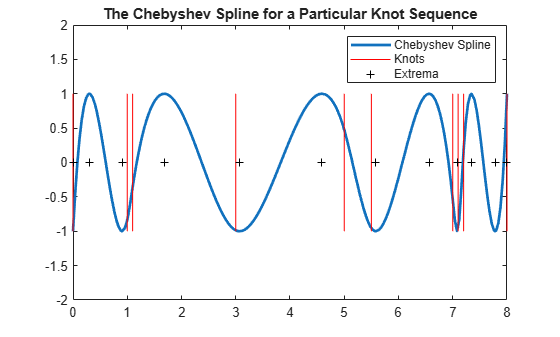# Construct Chebyshev Spline

This example shows how to use commands from Curve Fitting Toolbox™ to construct a Chebyshev spline.

### Chebyshev (a.k.a. Equioscillating) Spline Defined

By definition, for given knot sequence `t` of length `n+k`, `C = C_{t,k}` is the unique element of `S_{t,k}` of max-norm 1 that maximally oscillates on the interval `[t_k .. t_{n+1}]` and is positive near `t_{n+1}`. This means that there is a unique strictly increasing `tau` of length `n` so that the function `C` in `S_{k,t}` given by

` C(tau(i)) = (-1)^{n-i},`

for all `i`, has max-norm 1 on `[t_k .. t_{n+1}]`. This implies that

` tau(1) = t_k,`

` tau(n) = t_{n+1},`

and that

` t_i < tau(i) < t_{k+i},`

for all `i`. In fact,

` t_{i+1} <= tau(i) <= t_{i+k-1},`

for all `i`. This brings up the point that the knot sequence `t` is assumed to make such an inequality possible, which turns out to be equivalent to having all the elements of `S_{k,t}` continuous.

```t = augknt([0 1 1.1 3 5 5.5 7 7.1 7.2 8], 4 ); [tau,C] = chbpnt(t,4); xx = sort([linspace(0,8,201),tau]); plot(xx,fnval(C,xx),'LineWidth',2); hold on breaks = knt2brk(t); bbb = repmat(breaks,3,1); sss = repmat([1;-1;NaN],1,length(breaks)); plot(bbb(:), sss(:),'r'); hold off ylim([-2 2]); title('The Chebyshev Spline for a Particular Knot Sequence'); legend({'Chebyshev Spline' 'Knots'});```In short, the Chebyshev spline `C` looks just like the Chebyshev polynomial. It performs similar functions. For example, its extrema `tau` are particularly good sites to interpolate at from `S_{k,t}` since the norm of the resulting projector is about as small as can be.

```hold on plot(tau,zeros(size(tau)),'k+'); hold off legend({'Chebyshev Spline' 'Knots' 'Extrema'});```### Choice of Spline Space

In this example, we try to construct `C` for a given spline space.

We deal with cubic splines with simple interior knots, specified by

```k = 4; breaks = [0 1 1.1 3 5 5.5 7 7.1 7.2 8]; t = augknt(breaks, k)```
```t = 1×16 0 0 0 0 1.0000 1.1000 3.0000 5.0000 5.5000 7.0000 7.1000 7.2000 8.0000 8.0000 8.0000 8.0000 ```

thus getting a spline space of dimension

`n = length(t)-k`
```n = 12 ```

### Initial Guess

As our initial guess for the `tau`, we use the knot averages

` tau(i) = (t_{i+1} + ... + t_{i+k-1})/(k-1)`

recommended as good interpolation site choices, and plot the resulting first approximation to `C`.

`tau = aveknt(t,k)`
```tau = 1×12 0 0.3333 0.7000 1.7000 3.0333 4.5000 5.8333 6.5333 7.1000 7.4333 7.7333 8.0000 ```
```b = (-ones(1,n)).^(n-1:-1:0); c = spapi(t,tau,b); plot(breaks([1 end]),[1 1],'k', breaks([1 end]),[-1 -1],'k'); hold on fnplt(c,'r',1); hold off ylim([-2 2]); title('First Approximation to an Equioscillating Spline');```### Iteration

For the complete leveling, use the Remez algorithm. This means that we construct a new `tau` as the extrema of our current approximation, `c`, to `C` and try again.

To find the extrema, first calculate the derivative `Dc` of the current approximation `c`.

`Dc = fnder(c);`

Take the zeros of `Dc` using the `fnzeros` function.The zeros represent the extrema of the current approximation `c`. The result is the new guess for tau.

`tau(2:n-1) = mean(fnzeros(Dc))`
```tau = 1×12 0 0.2765 0.9057 1.7438 3.0779 4.5531 5.5830 6.5841 7.0809 7.3464 7.7889 8.0000 ```
```plot(breaks([1 end]),[1 1],'k', breaks([1 end]),[-1 -1],'k'); hold on fnplt(c,'r',1); plot(tau(2:n-1),zeros(1,n-2),'x'); hold off title('First Approximation to an Equioscillating Spline'); ax = gca; h = ax.Children; legend(h([2 1]),{'Approximation','Extrema'}); axis([0 8 -2 2]);```### End of First Iteration Step

We compute the resulting new approximation to the Chebyshev spline using the new guess for `tau`.

`cnew = spapi(t,tau,b);`

The new approximation is more nearly an equioscillating spline.

```plot(breaks([1 end]),[1 1],'k', breaks([1 end]),[-1 -1],'k'); hold on fnplt(c,'r',1); fnplt(cnew, 'k', 1); hold off ax = gca; h = ax.Children; legend(h([2 1]),{'First Approximation' 'Updated Approximation'}); axis([0 8 -2 2]);```If this is not close enough, simply try again, starting from this new `tau`. For this particular example, the next iteration already provides the Chebyshev spline to graphic accuracy.

### Use of Chebyshev-Demko Points

The Chebyshev spline for a given spline space `S_{k,t}`, along with its extrema, are available as optional outputs from the `chbpnt` command in the toolbox. These extrema were proposed as good interpolation sites by Steven Demko, hence are now called the Chebyshev-Demko sites. This section shows an example of their use.

If you have decided to approximate the square-root function on the interval `[0 .. 1]` by cubic splines with knot sequence

```k = 4; n = 10; t = augknt(((0:n)/n).^8,k);```

then a good approximation to the square-root function from that specific spline space is given by

```tau = chbpnt(t,k); sp = spapi(t,tau,sqrt(tau));```

as is evidenced by the near equioscillation of the error.

```xx = linspace(0,1,301); plot(xx, fnval(sp,xx)-sqrt(xx)); title({'Error in Interpolant to Square Root','at Chebyshev-Demko Sites.'});```